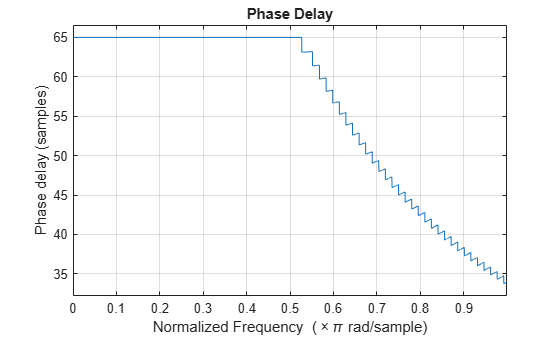# phasedelay

Phase delay response of discrete-time filter System object

## Syntax

``````[phi,w] = phasedelay(sysobj)``````
``````[phi,w] = phasedelay(sysobj,n)``````
``````[phi,w] = phasedelay(sysobj,n,fs)``````
``````[phi,w] = phasedelay(___,'Arithmetic',arithType)``````
``phasedelay(sysobj)``

## Description

``````[phi,w] = phasedelay(sysobj)``` returns the phase delay response vector, `phi`, and the corresponding frequency vector, `w`, in radians/sample of the filter System object™ based on the current filter coefficients.```
``````[phi,w] = phasedelay(sysobj,n)``` returns the `n`-point phase delay response vector and the corresponding `n`-point frequency vector in radians/sample of the filter System object.```
``````[phi,w] = phasedelay(sysobj,n,fs)``` uses `fs` to calculate the delay response. When you specify this syntax without any output arguments, the function launches `fvtool` and plots the response to `fs`/2.```
``````[phi,w] = phasedelay(___,'Arithmetic',arithType)``` analyzes the filter System object, based on the arithmetic specified in `arithType` using anyone of the previous syntaxes.```

example

````phasedelay(sysobj)` launches `fvtool` and shows the phase delay response of the filter System object.For more input options, see `phasedelay` in Signal Processing Toolbox™.```

## Examples

collapse all

Design an FIR filter.

```Fs = 8000; Fcutoff = 2000; FIRFilt = dsp.FIRFilter('Numerator', fir1(130,Fcutoff/(Fs/2)));```

The `phasedelay` function computes the phase delay of the filter and displays it using FVTool.

`phasedelay(FIRFilt)`## Input Arguments

collapse all

Number of points over which the phase response is evaluated around the upper half of the unit circle. For an FIR filter where `n` is a power of two, the computation is done faster using FFTs.

Data Types: `double`

Sampling frequency used in computing the phase delay response, specified as a positive scalar.

Data Types: `single` | `double`

Arithmetic used in the filter analysis, specified as `'double'`, `'single'`, or `'Fixed'`. When the arithmetic input is not specified and the filter System object is unlocked, the analysis tool assumes a double-precision filter. When the arithmetic input is not specified and the System object is locked, the function performs the analysis based on the data type of the locked input.

The `'Fixed'` value applies to filter System objects with fixed-point properties only.

When the `'Arithmetic'` input argument is specified as `'Fixed'` and the filter object has the data type of the coefficients set to `'Same word length as input'`, the arithmetic analysis depends on whether the System object is unlocked or locked.

• unlocked –– The analysis object function cannot determine the coefficients data type. The function assumes that the coefficients data type is signed, has a 16-bit word length, and is auto scaled. The function performs fixed-point analysis based on this assumption.

• locked –– When the input data type is `'double'` or `'single'`, the analysis object function cannot determine the coefficients data type. The function assumes that the data type of the coefficients is signed, has a 16-bit word length, and is auto scaled. The function performs fixed-point analysis based on this assumption.

To check if the System object is locked or unlocked, use the `isLocked` function.

When the arithmetic input is specified as `'Fixed'` and the filter object has the data type of the coefficients set to a custom numeric type, the object function performs fixed-point analysis based on the custom numeric data type.

## Output Arguments

collapse all

Phase delay response vector, evaluated at `n` equally-spaced points around the upper half of the unit circle, returned as a vector. If `n` is not specified, the function uses a default value of `8192`.

Data Types: `double`

Frequency vector of length `n` in radians/sample. `w` consists of `n` equally-spaced points around the upper half of the unit circle (from 0 to π radians/sample). If `n` is not specified, the function uses a default value of `8192`.

Data Types: `double`

## Version History

Introduced in R2011a